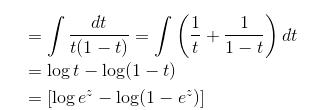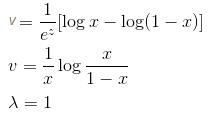Courses

# Differential Equation NAT Level - 2

## 10 Questions MCQ Test Topic wise Tests for IIT JAM Physics | Differential Equation NAT Level - 2

Description
This mock test of Differential Equation NAT Level - 2 for IIT JAM helps you for every IIT JAM entrance exam. This contains 10 Multiple Choice Questions for IIT JAM Differential Equation NAT Level - 2 (mcq) to study with solutions a complete question bank. The solved questions answers in this Differential Equation NAT Level - 2 quiz give you a good mix of easy questions and tough questions. IIT JAM students definitely take this Differential Equation NAT Level - 2 exercise for a better result in the exam. You can find other Differential Equation NAT Level - 2 extra questions, long questions & short questions for IIT JAM on EduRev as well by searching above.
*Answer can only contain numeric values
QUESTION: 1

### Find the orthogonal trajectory of the family of curves, y2 = x3 – 2 so, find the value of y as x → ∞.

Solution: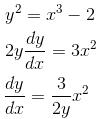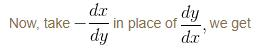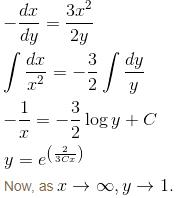*Answer can only contain numeric values
QUESTION: 2

### If the P.I. of the Differential Equation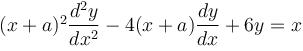is of the value form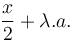Find the value of λ.

Solution:

This is an example of differential equation reducible to Homogeneous Equations.

Now, put  x + a = ez.
or   z = log(x + a)
Now,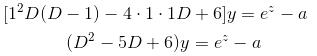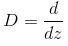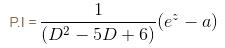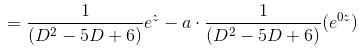⇒ λ = 1/3 = 0.333

*Answer can only contain numeric values
QUESTION: 3

### If the solution of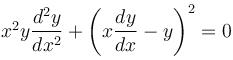is of the y2 = a1x + a2xα. Find the value of  α.

Solution: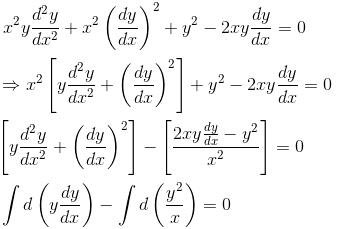Integrating Both sides, we get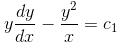Now, it is a Bernoulli's Equation, so we put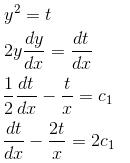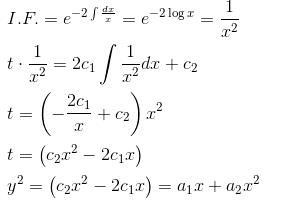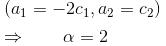*Answer can only contain numeric values
QUESTION: 4

If ex and x are solutions of Homogeneous equations (1 – x)y2 + xy1 – y = 2(x – 1)2e–x then the general solution y is of the form y = c1x + c2ex + α - xe-x.  Find the value of α.

Solution:

Let y1 = ex and y2 = x be two solutions of given Differential Equation. Thus,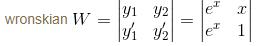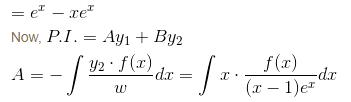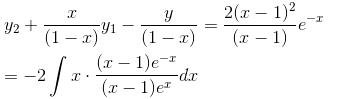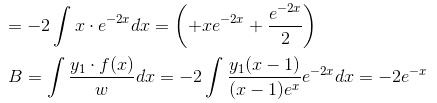By the method of variation of parameters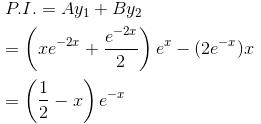Hence, α = 0.5

*Answer can only contain numeric values
QUESTION: 5

Find the order of differential equation of all tangents to parabola y2 = x

Solution: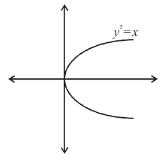Equation of parabola is

y2 = x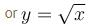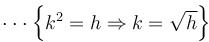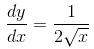At any point (hk) on parabola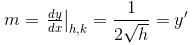Equation of tangent
(y – k) = m(x – h)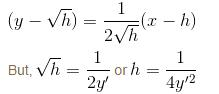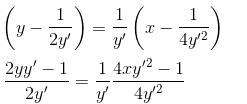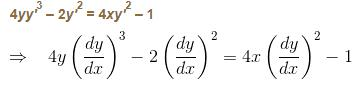Hence, order of differential equation = 1.

*Answer can only contain numeric values
QUESTION: 6

If the P.I. of the differential equation (D2 – 1)y = coshxcosx is of the form α.(2 sin hx sin x - cosx).

Solution: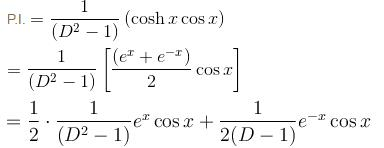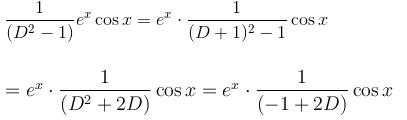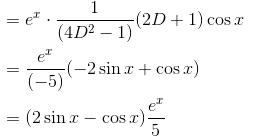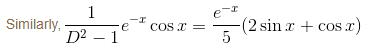Combining both, we get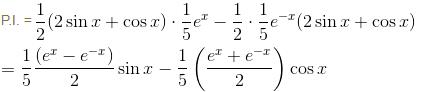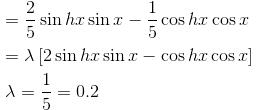*Answer can only contain numeric values
QUESTION: 7

An integrating factor of the equation dy/dx + x sin 2y = x3 cos2 y is f(x). Find the value of f(0).

Solution: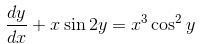Dividing complete equation by cos2y, we get.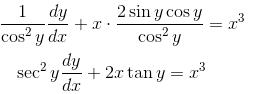Put tan y = t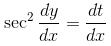This is Bernoulli’s equations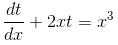Now, it is a linear Differential Equation.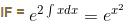∴   f(x) =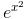f(0) = e0 = 1

*Answer can only contain numeric values
QUESTION: 8

If the PI of differential equation (D2 - 4D  + 4) y = 8x2e2x sin 2x is of the form eαx [(α'x2 + α") sin βx + β' x cos βx] then find the value of α".

Solution: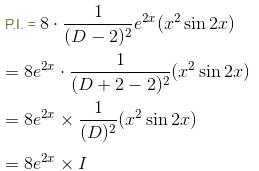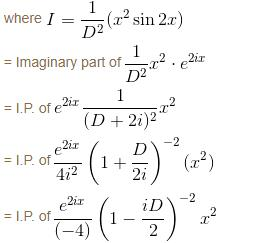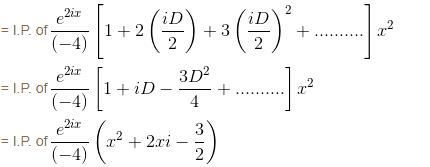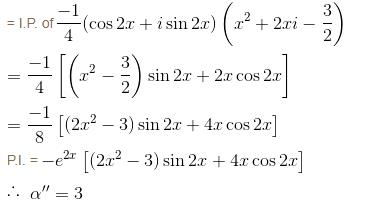*Answer can only contain numeric values
QUESTION: 9

If the integrating factor of the differential equation (x7y2 + 3y)dx + (3x8t - x)dy = 0 is of the form xmyn then sum of values of m and n is :

Solution:

Let the I.F be xmyn.

Multiplying Differential Equation by I.F it becomes exact

⇒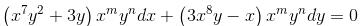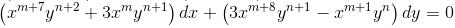This Differential Equation is Exact.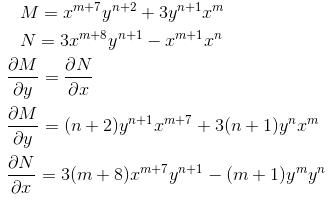It is exact

⇒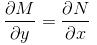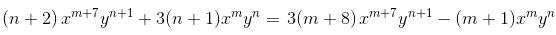3m - n = - 22
m + 3n = - 4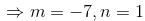m + n = –7 + 1  = –6

*Answer can only contain numeric values
QUESTION: 10

If the P.I. of Differential Equation (x2D2 + 3xD + 1) y = 1/(1 - x)2 is of form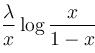then, find value of λ.

Solution: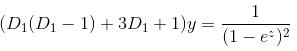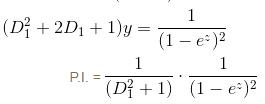Now, let us put.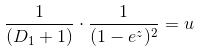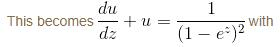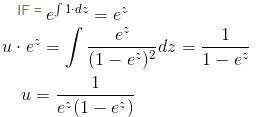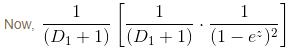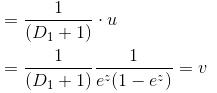Solving above Differential Equation, we get,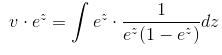Put ez = t
ezdz = dt